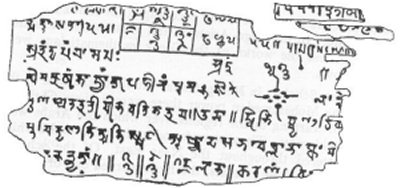A# Bakhshali manuscriptThe Bakhshali manuscript is an early mathematical manuscript, written on birch bark and found in the summer of 1881 near the village of Bakhshali in the Yusufzai subdivision of the Peshawar district (now in Pakistan). A large part of the manuscript had been destroyed and only about 70 leaves of birch bark, of which a few were mere scraps, survived to the time of its discovery. Although its date is uncertain, it is most commonly put at about the third or 4th century AD. and appears to be a commentary on an earlier mathematical work. Among the rules and techniques it sets out for solving problems, mostly in arithmetic and algebra, but also to a lesser extent in geometry and menstruation, is this formula (stated here in modern terms) for calculating the square root of a non-square number Q:

√Q = √(A2 + b) = A + b/2A - (b/2A)2/[2(A + b/2A)]

If Q = 41 (so that A = 6 and b = 5) this gives √Q = 6.403138528, which compares very favorably with the correct result of 6.403124237.